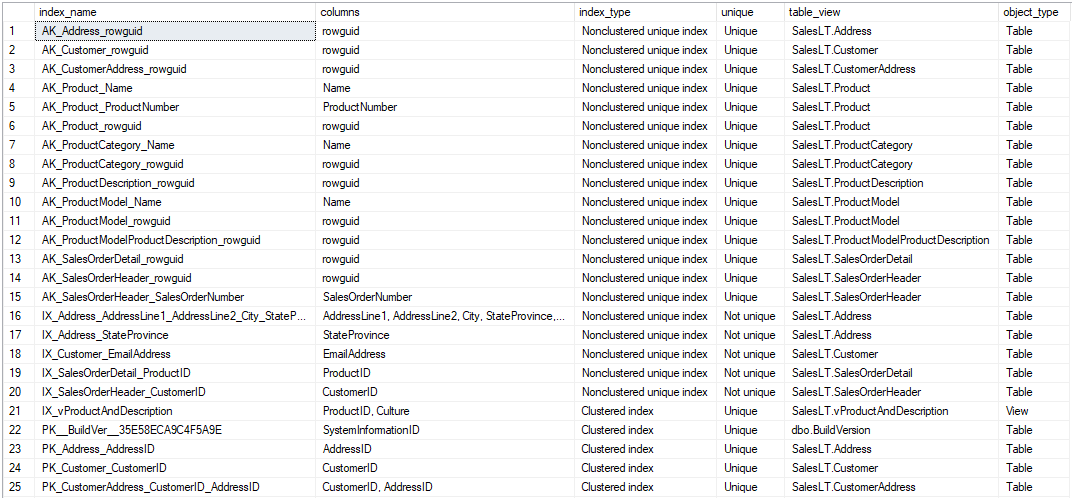## List all indexes in Azure SQL Database

Article for:

The query below lists all indexes in the database.

## Query

``````select i.[name] as index_name,
substring(column_names, 1, len(column_names)-1) as [columns],
case when i.[type] = 1 then 'Clustered index'
when i.[type] = 2 then 'Nonclustered unique index'
when i.[type] = 3 then 'XML index'
when i.[type] = 4 then 'Spatial index'
when i.[type] = 5 then 'Clustered columnstore index'
when i.[type] = 6 then 'Nonclustered columnstore index'
when i.[type] = 7 then 'Nonclustered hash index'
end as index_type,
case when i.is_unique = 1 then 'Unique'
else 'Not unique' end as [unique],
schema_name(t.schema_id) + '.' + t.[name] as table_view,
case when t.[type] = 'U' then 'Table'
when t.[type] = 'V' then 'View'
end as [object_type]
from sys.objects as t
inner join sys.indexes as i
on t.object_id = i.object_id
cross apply (select col.[name] + ', '
from sys.index_columns as ic
inner join sys.columns as col
on ic.object_id = col.object_id
and ic.column_id = col.column_id
where ic.object_id = t.object_id
and ic.index_id = i.index_id
order by col.column_id
for xml path ('') ) as D (column_names)
where t.is_ms_shipped <> 1
and index_id > 0
order by i.[name]
``````

## Columns

• index_name - index name
• columns - list of index columns separated with ","
• index_type
• Clustered index
• Nonclustered unique index
• XML index
• Spatial index
• Clustered columnstore index
• Nonclustered columnstore index
• Nonclustered hash index
• unique - whether index is unique
• Unique
• Not unique
• table_view - index table or view schema and name
• object_type - type of object index is defined for:
• Table
• View

## Rows

• One row represents one index
• Scope of rows: all indexes in the database
• Ordered by index name

## Sample results

Indexes in database.